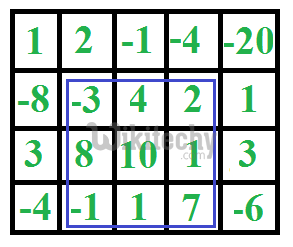# Maximum sum rectangle in a 2D matrix

Java Programming - Maximum sum rectangle in a 2D matrix - Dynamic Programming - Given a 2D array, find the maximum sum subarray in it. For example

Given a 2D array, find the maximum sum subarray in it. For example, in the following 2D array, the maximum sum subarray is highlighted with blue rectangle and sum of this subarray is 29.This problem is mainly an extension of Largest Sum Contiguous Subarray for 1D array.

The naive solution for this problem is to check every possible rectangle in given 2D array. This solution requires 4 nested loops and time complexity of this solution would be O(n^4).

Kadane’s algorithm for 1D array can be used to reduce the time complexity to O(n^3). The idea is to fix the left and right columns one by one and find the maximum sum contiguous rows for every left and right column pair. We basically find top and bottom row numbers (which have maximum sum) for every fixed left and right column pair.

To find the top and bottom row numbers, calculate sum of elements in every row from left to right and store these sums in an array say temp[]. So temp[i] indicates sum of elements from left to right in row i. If we apply Kadane’s 1D algorithm on temp[], and get the maximum sum subarray of temp, this maximum sum would be the maximum possible sum with left and right as boundary columns. To get the overall maximum sum, we compare this sum with the maximum sum so far.

### Java implementation for Maximum sum rectangle in a 2D matrix:

Java
``````import java.util.*;
import java.lang.*;
import java.io.*;

class Ideone
{
public static void main (String[] args) throws java.lang.Exception
{
findMaxSubMatrix(new int[][] {
{1, 2, -1, -4, -20},
{-8, -3, 4, 2, 1},
{3, 8, 10, 1, 3},
{-4, -1, 1, 7, -6}
});
}

public static int[] kadane(int[] a) {
int[] result = new int[]{Integer.MIN_VALUE, 0, -1};
int currentSum = 0;
int localStart = 0;

for (int i = 0; i < a.length; i++) {
currentSum += a[i];
if (currentSum < 0) {
currentSum = 0;
localStart = i + 1;
} else if (currentSum > result) {
result = currentSum;
result = localStart;
result = i;
}
}

if (result == -1) {
result = 0;
for (int i = 0; i < a.length; i++) {
if (a[i] > result) {
result = a[i];
result = i;
result = i;
}
}
}

return result;
}

public static void findMaxSubMatrix(int[][] a) {
int cols = a.length;
int rows = a.length;
int[] currentResult;
int maxSum = Integer.MIN_VALUE;
int left = 0;
int top = 0;
int right = 0;
int bottom = 0;

for (int leftCol = 0; leftCol < cols; leftCol++) {
int[] tmp = new int[rows];

for (int rightCol = leftCol; rightCol < cols; rightCol++) {

for (int i = 0; i < rows; i++) {
tmp[i] += a[i][rightCol];
}
if (currentResult > maxSum) {
maxSum = currentResult;
left = leftCol;
top = currentResult;
right = rightCol;
bottom = currentResult;
}
}
}
System.out.println("MaxSum: " + maxSum +
", range: [(" + left + ", " + top +
")(" + right + ", " + bottom + ")]");
}
}``````

### Output :

`Max sum is: 29, range: [(1,1)(3,3]`

Time Complexity: O(n^3)

READ  C Programming - Program for Fibonacci numbers#### Venkatesan Prabu

Wikitechy Founder, Author, International Speaker, and Job Consultant. My role as the CEO of Wikitechy, I help businesses build their next generation digital platforms and help with their product innovation and growth strategy. I'm a frequent speaker at tech conferences and events.